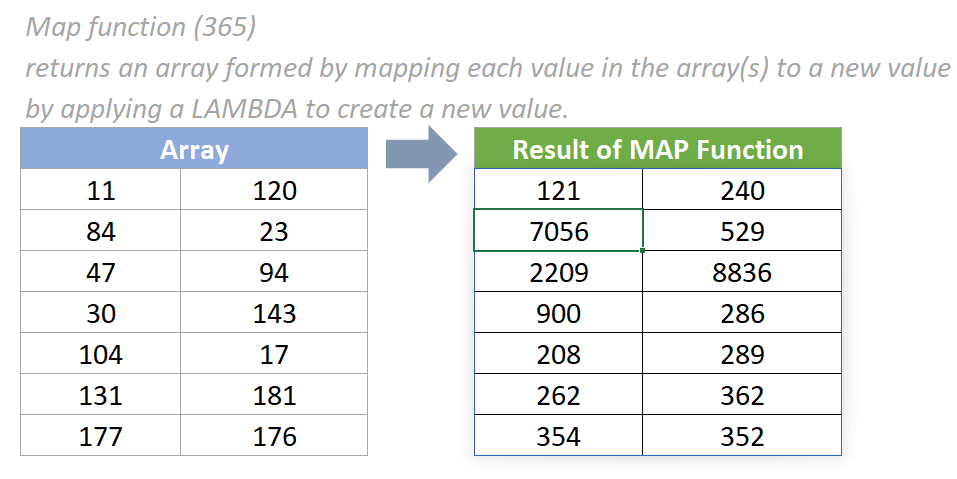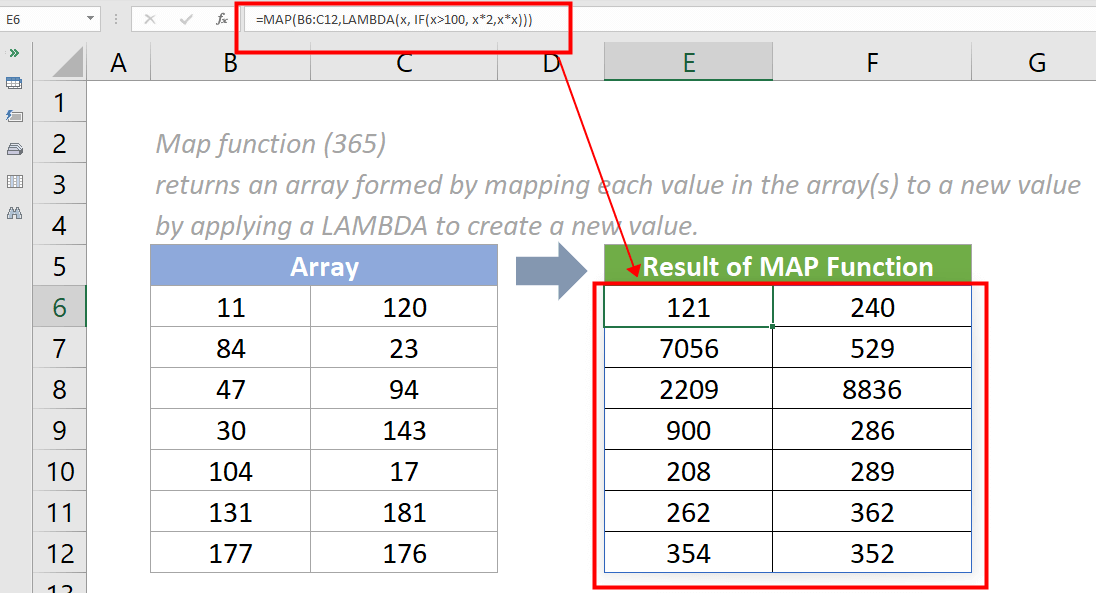## Excel MAP function (365)

The MAP function applies a LAMBDA function to create a new value and returns an array formed by mapping each value in the supplied array(s) to a new value.#### Syntax

=MAP (array1,[array2],...,lambda)

#### Arguments

• Array1 (required): The array to be mapped.
• Array2,... (optional): Additional arrays to be mapped.
• Lambda (required): The custom LAMBDA function applies to the array(s).
It must be the last argument and configured to accept additional parameters while additional arrays are provided.

#### Return Value

The MAP function returns arrays of results.

#### Function notes

1. The MAP function is newly introduced in Excel for Microsoft 365. So it is not available in earlier versions of Excel. New dynamic array formulas are introduced in Excel for Microsoft 365, meaning no need to use Ctrl+ Shift+ Enter to enter the MAP formula as an array formula.
2. The #VALUE! error value occurs if one of the below situations occur:
• an invalid LAMBDA function is provided;
• a wrong number of parameters is provided.

#### Example

As the below screenshot shows, there is an array of data. To multiply the number in this array by 2 when it is larger than 100 and to square this number when it is smaller or equal to 100, please do as follows:

Please copy the formula below into cell E6, then press the Enter key to get the result.

=MAP (B6:C12,LAMBDA(x, IF(x>100, x*2,x*x)))#### Relative Functions:

• Excel BYROW Function
The Excel BYROW function applies a LAMBDA function to each row in a given array and returns the result per row as a single array.

• Excel LAMBDA Function
The Excel LAMBDA function is used to create custom functions that can be reused throughout a workbook.

• Excel MAKEARRAY Function
The Excel MAKEARRAY function returns a calculated array based on the given number of rows and columns.